# Ap review total

1 de May de 2012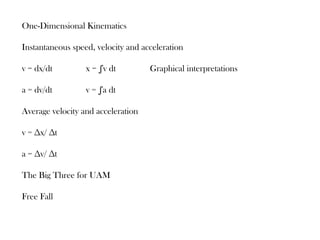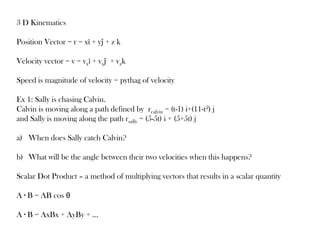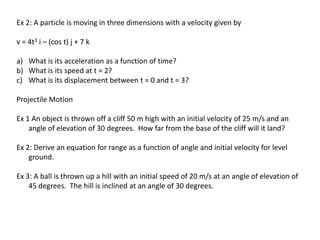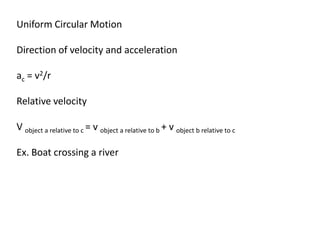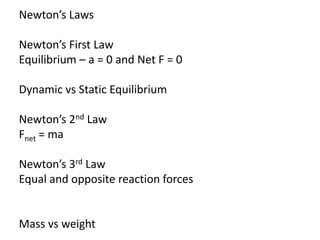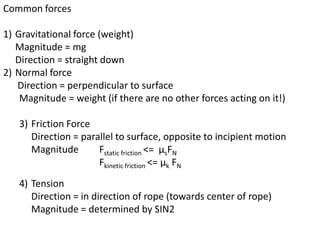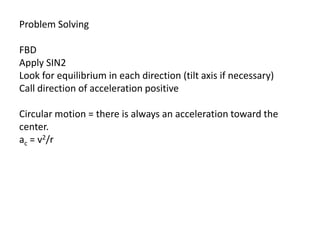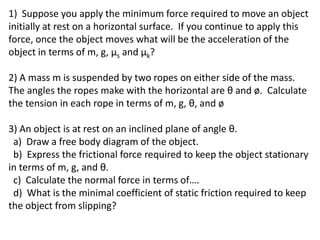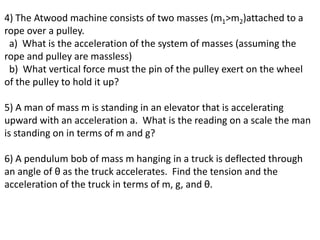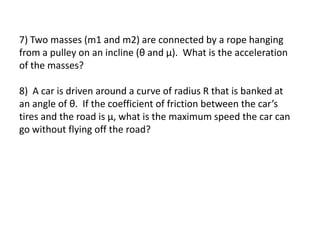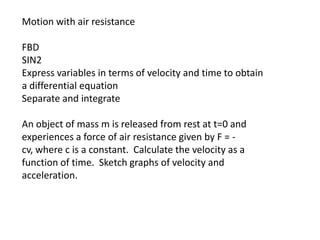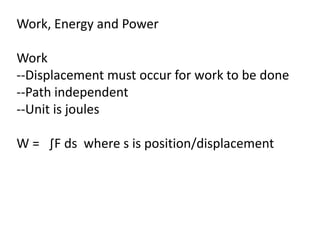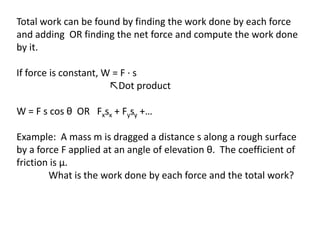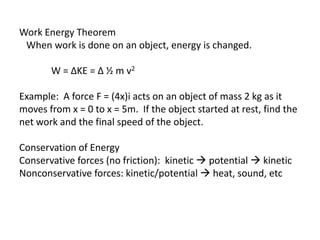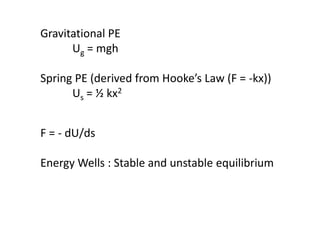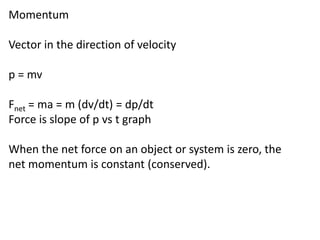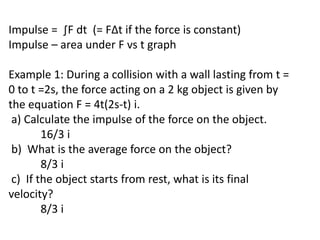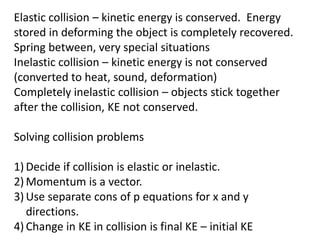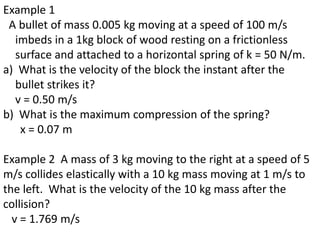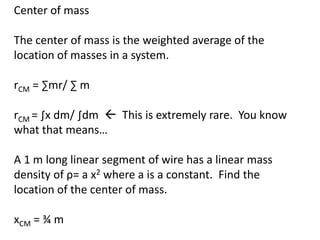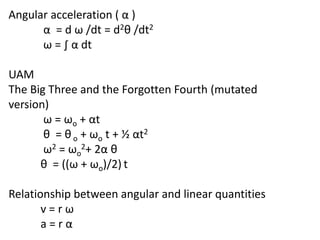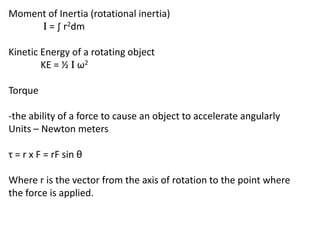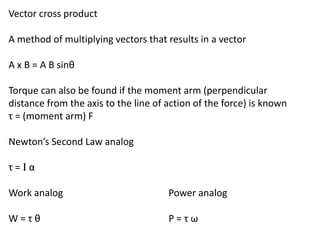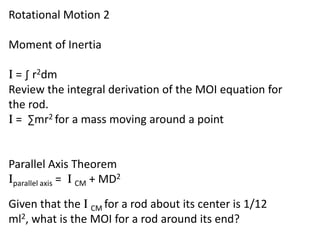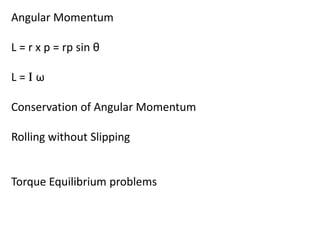1 de 27

### Ap review total

• 1. One-Dimensional Kinematics Instantaneous speed, velocity and acceleration v = dx/dt x = ∫v dt Graphical interpretations a = dv/dt v = ∫a dt Average velocity and acceleration v = ∆x/ ∆t a = ∆v/ ∆t The Big Three for UAM Free Fall
• 2. 3 D Kinematics Position Vector = r = xî + yĵ + z k Velocity vector = v = vxî + vyĵ + vzk Speed is magnitude of velocity = pythag of velocity Ex 1: Sally is chasing Calvin. Calvin is moving along a path defined by rcalvin = (t-1) i+(11-t2) j and Sally is moving along the path rsally = (5-5t) i + (5+5t) j a) When does Sally catch Calvin? b) What will be the angle between their two velocities when this happens? Scalar Dot Product – a method of multiplying vectors that results in a scalar quantity A ∙ B = AB cos θ A ∙ B = AxBx + AyBy + …
• 3. Ex 2: A particle is moving in three dimensions with a velocity given by v = 4t3 i – (cos t) j + 7 k a) What is its acceleration as a function of time? b) What is its speed at t = 2? c) What is its displacement between t = 0 and t = 3? Projectile Motion Ex 1 An object is thrown off a cliff 50 m high with an initial velocity of 25 m/s and an angle of elevation of 30 degrees. How far from the base of the cliff will it land? Ex 2: Derive an equation for range as a function of angle and initial velocity for level ground. Ex 3: A ball is thrown up a hill with an initial speed of 20 m/s at an angle of elevation of 45 degrees. The hill is inclined at an angle of 30 degrees.
• 4. Uniform Circular Motion Direction of velocity and acceleration ac = v2/r Relative velocity V object a relative to c = v object a relative to b + v object b relative to c Ex. Boat crossing a river
• 5. Newton’s Laws Newton’s First Law Equilibrium – a = 0 and Net F = 0 Dynamic vs Static Equilibrium Newton’s 2nd Law Fnet = ma Newton’s 3rd Law Equal and opposite reaction forces Mass vs weight
• 6. Common forces 1) Gravitational force (weight) Magnitude = mg Direction = straight down 2) Normal force Direction = perpendicular to surface Magnitude = weight (if there are no other forces acting on it!) 3) Friction Force Direction = parallel to surface, opposite to incipient motion Magnitude Fstatic friction <= µsFN Fkinetic friction <= µk FN 4) Tension Direction = in direction of rope (towards center of rope) Magnitude = determined by SIN2
• 7. Problem Solving FBD Apply SIN2 Look for equilibrium in each direction (tilt axis if necessary) Call direction of acceleration positive Circular motion = there is always an acceleration toward the center. ac = v2/r
• 8. 1) Suppose you apply the minimum force required to move an object initially at rest on a horizontal surface. If you continue to apply this force, once the object moves what will be the acceleration of the object in terms of m, g, µs and µk? 2) A mass m is suspended by two ropes on either side of the mass. The angles the ropes make with the horizontal are θ and ø. Calculate the tension in each rope in terms of m, g, θ, and ø 3) An object is at rest on an inclined plane of angle θ. a) Draw a free body diagram of the object. b) Express the frictional force required to keep the object stationary in terms of m, g, and θ. c) Calculate the normal force in terms of…. d) What is the minimal coefficient of static friction required to keep the object from slipping?
• 9. 4) The Atwood machine consists of two masses (m1>m2)attached to a rope over a pulley. a) What is the acceleration of the system of masses (assuming the rope and pulley are massless) b) What vertical force must the pin of the pulley exert on the wheel of the pulley to hold it up? 5) A man of mass m is standing in an elevator that is accelerating upward with an acceleration a. What is the reading on a scale the man is standing on in terms of m and g? 6) A pendulum bob of mass m hanging in a truck is deflected through an angle of θ as the truck accelerates. Find the tension and the acceleration of the truck in terms of m, g, and θ.
• 10. 7) Two masses (m1 and m2) are connected by a rope hanging from a pulley on an incline (θ and µ). What is the acceleration of the masses? 8) A car is driven around a curve of radius R that is banked at an angle of θ. If the coefficient of friction between the car’s tires and the road is µ, what is the maximum speed the car can go without flying off the road?
• 11. Motion with air resistance FBD SIN2 Express variables in terms of velocity and time to obtain a differential equation Separate and integrate An object of mass m is released from rest at t=0 and experiences a force of air resistance given by F = - cv, where c is a constant. Calculate the velocity as a function of time. Sketch graphs of velocity and acceleration.
• 12. Work, Energy and Power Work --Displacement must occur for work to be done --Path independent --Unit is joules W = ∫F ds where s is position/displacement
• 13. Total work can be found by finding the work done by each force and adding OR finding the net force and compute the work done by it. If force is constant, W = F ∙ s ↖Dot product W = F s cos θ OR Fxsx + Fysy +… Example: A mass m is dragged a distance s along a rough surface by a force F applied at an angle of elevation θ. The coefficient of friction is µ. What is the work done by each force and the total work?
• 14. Work Energy Theorem When work is done on an object, energy is changed. W = ∆KE = ∆ ½ m v2 Example: A force F = (4x)i acts on an object of mass 2 kg as it moves from x = 0 to x = 5m. If the object started at rest, find the net work and the final speed of the object. Conservation of Energy Conservative forces (no friction): kinetic  potential  kinetic Nonconservative forces: kinetic/potential  heat, sound, etc
• 15. Gravitational PE Ug = mgh Spring PE (derived from Hooke’s Law (F = -kx)) Us = ½ kx2 F = - dU/ds Energy Wells : Stable and unstable equilibrium
• 16. Power Rate at which work is done P = W/t Of course, any equation can be turned into a calculus version: P = dW/dt = F v cos θ
• 17. Momentum Vector in the direction of velocity p = mv Fnet = ma = m (dv/dt) = dp/dt Force is slope of p vs t graph When the net force on an object or system is zero, the net momentum is constant (conserved).
• 18. Impulse = ∫F dt (= F∆t if the force is constant) Impulse – area under F vs t graph Example 1: During a collision with a wall lasting from t = 0 to t =2s, the force acting on a 2 kg object is given by the equation F = 4t(2s-t) i. a) Calculate the impulse of the force on the object. 16/3 i b) What is the average force on the object? 8/3 i c) If the object starts from rest, what is its final velocity? 8/3 i
• 19. Elastic collision – kinetic energy is conserved. Energy stored in deforming the object is completely recovered. Spring between, very special situations Inelastic collision – kinetic energy is not conserved (converted to heat, sound, deformation) Completely inelastic collision – objects stick together after the collision, KE not conserved. Solving collision problems 1) Decide if collision is elastic or inelastic. 2) Momentum is a vector. 3) Use separate cons of p equations for x and y directions. 4) Change in KE in collision is final KE – initial KE
• 20. Example 1 A bullet of mass 0.005 kg moving at a speed of 100 m/s imbeds in a 1kg block of wood resting on a frictionless surface and attached to a horizontal spring of k = 50 N/m. a) What is the velocity of the block the instant after the bullet strikes it? v = 0.50 m/s b) What is the maximum compression of the spring? x = 0.07 m Example 2 A mass of 3 kg moving to the right at a speed of 5 m/s collides elastically with a 10 kg mass moving at 1 m/s to the left. What is the velocity of the 10 kg mass after the collision? v = 1.769 m/s
• 21. Center of mass The center of mass is the weighted average of the location of masses in a system. rCM = ∑mr/ ∑ m rCM = ∫x dm/ ∫dm  This is extremely rare. You know what that means… A 1 m long linear segment of wire has a linear mass density of ρ= a x2 where a is a constant. Find the location of the center of mass. xCM = ¾ m
• 22. Rotational Motion 1 Fixed Axis rotation θ = angular position = arc length / radius measured in radians 1 revolution = 2π radians = 360 degrees Angular velocity ( ω ) measured in radians/second ω = dθ /dt θ = ∫ω dt
• 23. Angular acceleration ( α ) α = d ω /dt = d2θ /dt2 ω = ∫ α dt UAM The Big Three and the Forgotten Fourth (mutated version) ω = ωo + αt θ = θ o + ωo t + ½ αt2 ω2 = ωo2+ 2α θ θ = ((ω + ωo)/2) t Relationship between angular and linear quantities v=rω a=rα
• 24. Moment of Inertia (rotational inertia) I = ∫ r2dm Kinetic Energy of a rotating object KE = ½ I ω2 Torque -the ability of a force to cause an object to accelerate angularly Units – Newton meters τ = r x F = rF sin θ Where r is the vector from the axis of rotation to the point where the force is applied.
• 25. Vector cross product A method of multiplying vectors that results in a vector A x B = A B sinθ Torque can also be found if the moment arm (perpendicular distance from the axis to the line of action of the force) is known τ = (moment arm) F Newton’s Second Law analog τ=Iα Work analog Power analog W=τθ P=τω
• 26. Rotational Motion 2 Moment of Inertia I = ∫ r2dm Review the integral derivation of the MOI equation for the rod. I = ∑mr2 for a mass moving around a point Parallel Axis Theorem Iparallel axis = I CM + MD2 Given that the I CM for a rod about its center is 1/12 ml2, what is the MOI for a rod around its end?
• 27. Angular Momentum L = r x p = rp sin θ L=Iω Conservation of Angular Momentum Rolling without Slipping Torque Equilibrium problems